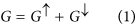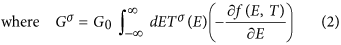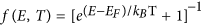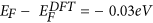## Introduction

Porphyrins offer a variety of desirable features as building blocks for future molecular-scale devices including their highly-conjugated structure, rigid planar geometry, high chemical stability and their ability to form metalloporphyrins by coordinating metal ions in the center of their macrocyclic and aromatic skeleton1,2,3,4,5. Following early work, which established their chemical and biological properties6,7,8,9, porphyrins have become a focus of interest both for experimental and theoretical investigations of molecular electronics10,11,12 and for the design of complexes using supramolecular chemistry, leading to a diverse array of structures available for nano-scale building blocks13. This unique combination of properties is exploited in nature, where for example metalloporphyrins acts as charge carriers in naturally occurring processes such as photosynthesis14,15,16,17 and in the respiratory chain18,19. In many of these processes, the plane of the porphyrin skeleton is stacked perpendicular to the direction of charge transport, whereas previous studies10,11,12 address conductance with the plane of the porphyrin skeleton aligned parallel to the direction of charge transport. In the latter “current in plane” (CIP) up-right configuration (Fig. 1a), the porphyrin skeleton was contacted to gold electrodes via thiol or pyridyl anchor groups and the electrical conductance was found to be low10,20 (of order nanosiemens). For the purpose of developing future single-molecule electronics and thermoelectrics, it is highly desirable to increase the electrical conductance, since this can lead to higher switching speeds and reduce the relative effect of parasitic phonons in thermoelectric devices. In what follows we develop a strategy for increasing the electrical conductance of porphyrin-based single-molecule wires by investigating their conductance with the current perpendicular to the plane (CPP) (Fig. 1b). We report a joint experimental and theoretical study of CPP conductance trends and binding configurations across a family of 5,15-diphenylporphyrins (DPPs), with a centrally-coordinated divalent metal ion of either Co(II), Ni, Cu or Zn and demonstrate that their conductance and stability can be tuned through the choice of metal atom. This is an extension of previous experimental measurement21 which showed that the CPP conductance of the flat-laying sandwiches of a Co(II)-DPP shows a large conductance value of three orders of magnitude higher than the measured in-plane conductance10.

## Results and Discussion

### Binding energies and relaxed configurations

To obtain theoretical results for binding energies and relaxed configurations, spin-polarised DFT calculations were carried out using SIESTA22 with the local density functional approximation parameterised by Ceperley and Adler23. Initially the geometry of each isolated porphyrin was optimised to a force tolerance less than 20 meV/Å using an extended double zeta polarised basis set of pseudo atomic orbitals for all atoms, and a mesh cutoff of 200 Ry to define the real space grid. Next, the binding energy EBof a single pyridine-4-yl-methanol (PY) with the porphyrin was calculated using the counterpoise method24,25 (see SI). For all four metallo-porphyrins, we find that the energetically-most-favorable configuration occurs when the PY nitrogen atoms are located above the metal atom of the porphyrin. For this most-favorable position of the PY nitrogen atoms, the results for all four binding energies and the corresponding nitrogen-metal distances are shown in Table 1.

### Conductance measurements

The Co-DPP and Zn-DPP molecules were synthesized according to the procedure described by Song et al.26. The synthesis of Cu-DPP and Ni-DPP described by26 was modified by changing the solvent and reaction times (See SI for experimental details). PY was synthesized as described previously by Puigmarti-Luis et al.27 and the single-molecule transport measurements were conducted following the procedure described in ref. 21.

Briefly, an Au(111) surface and a STM Au-electrode tip were both functionalized with 1 mM PY solution in ethanol and incubated for 24 h (See Experiments SI for further details). Both electrodes were mounted onto the STM cell and the cell was filled with mesitylene, an inert, non-polar organic solvent, in which the target M(II)-porphyrin is solubilized in nano-molar concentrations25. Details about the STM-break junction (STM-BJ) measurements can be found elsewhere (see SI). Briefly, the STM-BJ experiments consist of repeatedly approaching and retracting the two pyridyl-functionalized electrodes, while monitoring the tunneling current flowing through the electrode-electrode STM junction under a low applied voltage bias (±10 and ±25 mV). ~5000 current traces were collected and 10–15% of them were used to build a conductance 1D histogram for each molecule, examples of which are shown in Fig. 2 insets. As control experiments, single-molecule conductance experiments in the absence of porphyrin molecules and in the presence of empty DPP were performed under the same experimental conditions (see details in Supporting Information). The absence of porphyrin molecules resulted in the lack of molecular junction events and the DPP showed no high conductance peak in the histograms. The fit of the observed high conductance peak in Fig. 2 histogram was used to extract a most-probable value of the single-molecule conductance for the flat-stacked metalloporphyrin21. The observed two low conductance peaks are commonly-observed for all porphyrins and they have been ascribed to molecular wires with more extended (tilted) conformations of the porphyrin bridging the gap at longer electrode-electrode separations25. The fact that the empty DDP uniquely displays the low conductance features is evidence that such conformations arise from the interaction between the PY and the porphyrin ring moieties. The conductance values extracted from Gaussian fits to the conductance histograms for each metalloporphyrin (Fig. 2) has been also supported by a static blinking STM approach, where the spontaneous formation of the porphyrin bridge is attained while holding a fixed electrode-electrode distance (see SI for more details on the blinking method).

### Conductance calculations

To model an example of a blinking experiment in which the electrodes are held at a fixed separation, we fixed the PY-functionalised gold electrodes at separation corresponding to a 4.6 Å distance between the terminal N atoms of the PYs, as shown in Fig. 3. This distance is chosen to be slightly larger than the highest value of the distances d in table 1, such that all molecules can be accommodated within the electrode gap. We then allowed the porphyrin molecule to bind to the lower PY, with a N-to-metal-atom distance of d (see Table 1). The PY of the upper gold electrode was therefore more weakly bound to the metal atom of the porphyrin, as would be the case in a blinking experiment.

Before computing transport properties, we first examined the spin state of the metalloporphyrins. Numerous studies have examined the effect of the axial ligand on the redox28,29 and photovoltaic properties of metalloporphyrins30. Nickel porphyrin with coordinating axial ligands are paramaganetic (S = 1) in contrast to four-coordinate species (S = 0)31,32. Therefore, to accurately calculate the transport properties of these molecules spin polarized transport calculations must be carried out. We find in the case of the zinc-metalloporphyrin were there is no spin dependence the up spin and down spin transmission curves are almost identical (See Fig. S1).

The conductance was then calculated using the Gollum quantum transport code33, which utilizes the mean-field Hamiltonians provided by DFT. Starting from the SIESTA Hamiltonian, we use Gollum to calculate the transmission coefficient Tσ(E), describing electrons of energy E, spin σ = [↑, ↓] passing from one electrode to the other via the porphyrin, from which the finite-temperature electrical conductance G is obtained using the Landauer formulaIn this expression, f(E, T) is the Fermi distribution function defined aswhere kB is Boltzmann’s constant andis the quantum of conductance.

Figure 4 shows the total transmission coefficients as a function of energy for Zn-DPP, Cu-DPP, Co-DPP and Ni-DPP respectively. The corresponding room-temperature conductances versus Fermi energy EF are shown in Fig. 5a. Since the Fermi energypredicted by DFT is not necessarily accurate34, to compare theory with experiment, we treat the Fermi energy EF as a single free parameter, chosen to determine four conductances, which are closest to the experimental trend. Figure 5b shows that the experimentally-measured order Ni < Co < Cu < Zn is obtained by choosing a Fermi energy.